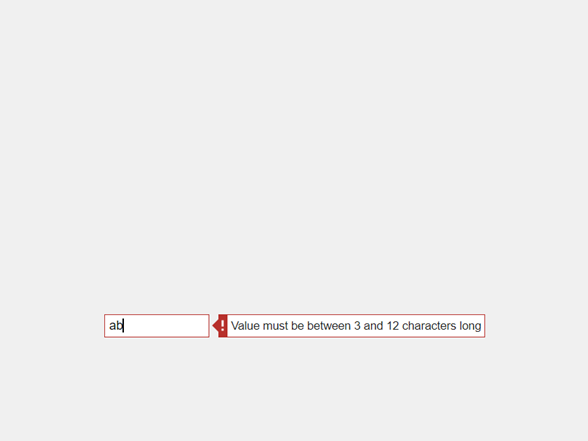# uieditfield

## 语法

``edt = uieditfield``
``edt = uieditfield(style)``
``edt = uieditfield(parent)``
``edt = uieditfield(parent,style)``
``edt = uieditfield(___,Name,Value)``

## 说明

``edt = uieditfield` 在新图窗窗口中创建一个文本编辑字段，并返回 `EditField` 对象。MATLAB® 调用 `uifigure` 函数来创建该图窗。`
``edt = uieditfield(style)` 创建指定样式的编辑字段。`

``edt = uieditfield(parent)` 在指定的父容器中创建编辑字段。父容器可以是使用 `uifigure` 函数创建的 `Figure` 或其子容器之一。`

``edt = uieditfield(parent,style)` 在指定的父容器中创建指定样式的编辑字段。`

``edt = uieditfield(___,Name,Value)` 使用一个或多个名称-值参数指定对象属性。可将此选项与上述语法中的任何输入参数组合一起使用。`

## 示例

```fig = uifigure; edt = uieditfield(fig);``````fig = uifigure; edt = uieditfield(fig,"numeric");``````fig = uifigure; pnl = uipanel(fig); edt = uieditfield(pnl,"numeric");``````fig = uifigure; edt = uieditfield(fig,"numeric", ... "RoundFractionalValues","on");```

`limits = edt.Limits`
```limits = -Inf Inf```

`edt.Limits = [0 100];`

```fig = uifigure; edt = uieditfield(fig,"numeric", ... "Limits",[-5 10], ... "LowerLimitInclusive","off", ... "UpperLimitInclusive","on", ... "Value",5);```

```fig = uifigure; edt = uieditfield(fig,"numeric", ... "ValueDisplayFormat","%.2f Volts");```

MATLAB 将值存储为 `5.5556`。如果您在编辑字段中再次点击，它会显示 `5.5556`。有关支持的格式化显示操作符的完整列表，请参阅 `sprintf`

```fig = uifigure; edt = uieditfield(fig, ... "CharacterLimits",[3 12], ... "InputType","alphanumerics");``````function textValue % Create figure and components. fig = uifigure("Position",[100 100 366 270]); lbl = uilabel(fig,... "Position",[130 100 100 15]); txt = uieditfield(fig,... "Position",[100 175 100 22],... "ValueChangedFcn",@(txt,event) textChanged(txt,lbl)); end % Code the callback function. function textChanged(txt,lbl) lbl.Text = txt.Value; end``````function numericEditFieldValue % Create figure and components fig = uifigure("Position",[100 100 366 270]); slider = uislider(fig,... "Position",[100 140 120 3]); numfld = uieditfield(fig,"numeric",... "Position",[110 200 100 22],... "ValueChangedFcn",@(numfld,event) numberChanged(numfld,slider)); end % Create ValueChangedFcn callback function numberChanged(numfld,slider) slider.Value = numfld.Value; end``````function logNames % Create figure and components fig = uifigure("Position",[100 100 366 400]); loglist = uitextarea(fig,... "Position",[134 49 150 277],... "Editable","off"); namefld = uieditfield(fig,"text",... "Value", "Bob Langley",... "Position",[134 367 100 22],... "ValueChangedFcn",@(namefld,event) nValChanged(namefld,event,loglist)); end % Create ValueChangedFcn callback function nValChanged(namefld,event,loglist) newvalue = event.Value; previousValue = event.PreviousValue; loglist.Value = [previousValue; loglist.Value]; end```## 输入参数

• `"text"`

默认情况下，文本编辑字段为空。

• `"numeric"`

默认情况下，数值编辑字段显示值 0。如果 App 用户在数值编辑字段中键入非数字值，MATLAB 将显示错误工具提示，并将值还原为上一个有效值。

### 名称-值参数

`EditField``NumericEditField` 对象支持不同的属性集合。有关对象的属性和描述的完整列表，请参阅相关联的属性页。